# MCQ in AC Circuits Part 3 | REE Board Exam

(Last Updated On: November 13, 2020)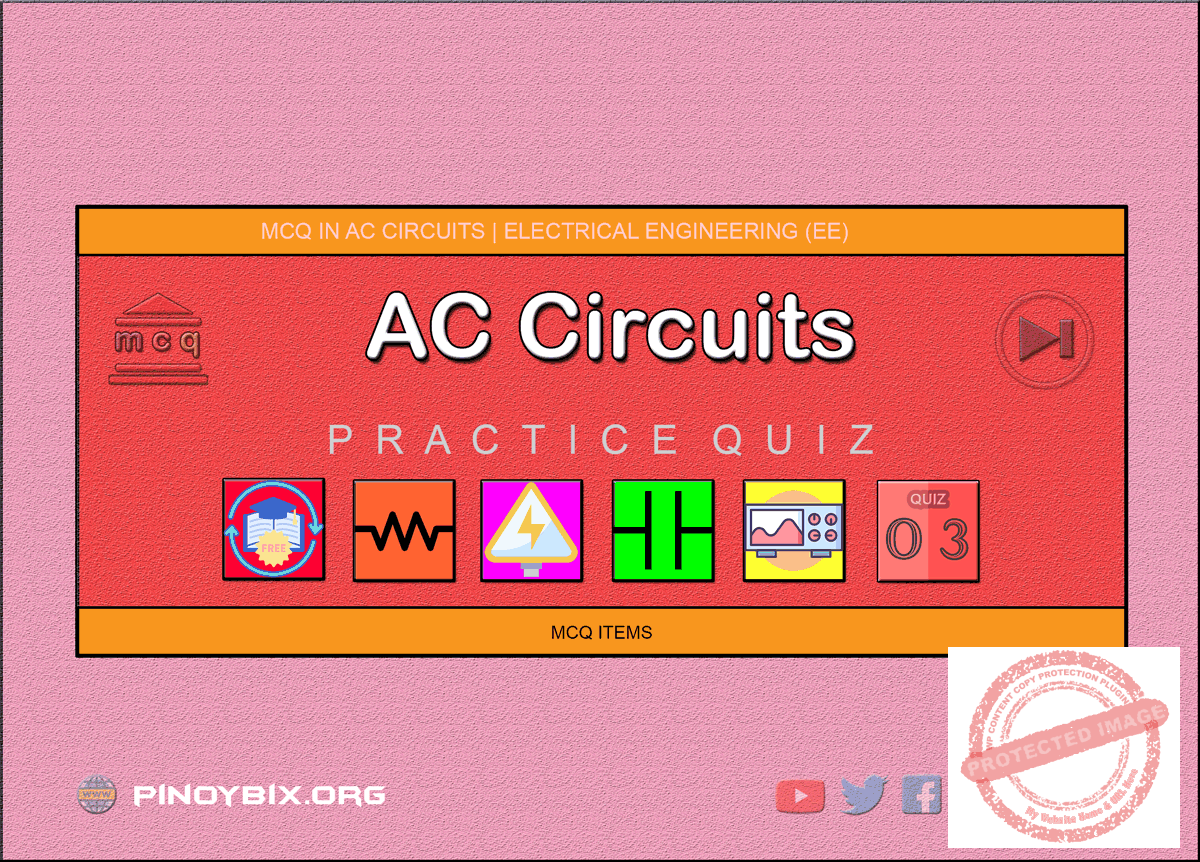This is the Multiple Choice Questions Part 3 of the Series in AC Circuits as one of the Electrical Engineering topic. In Preparation for the REE Board Exam make sure to expose yourself and familiarize in each and every questions compiled here taken from various sources including but not limited to past Board Exam Questions in Electrical Engineering field, Electrical Engineering Books, Journals and other Electrical Engineering References.

#### Continue Practice Exam Test Questions Part 3 of the Series

SINUSOIDAL VOLTAGE AND CURRENT

Choose the letter of the best answer in each questions.

101.  Each of the three coils generates an e.m.f. of 230 V. The e.m.f. of second leads  that  of  the  first  120 V  and  the  third  lags  behind  the  first  by  the  same angle. The resultant e.m.f. across the series combination of the coils is ______.

A.  0 V

B.  230 V

C.  690 V

D.  none of these

Solution:

102.  In Fig. 1.4, I1 + I2 is equal to ____.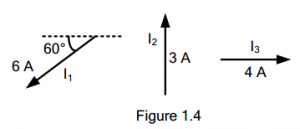A.   3 A

B.  4.33 A

C.  9 A

D.  3.43 A

Solution:

103.  In Fig. 1.4, I2 + I3 is equal to _____.A.  7 A

B.  √13   A

C.  5 A

D.  none of these

Solution:

104.  In Fig. 1.5, E1 + E2 + E3 + E4 is equal to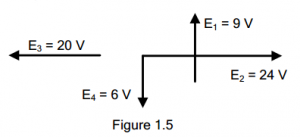A.  7 V

B.  5 V

C.  20 V

D.  none of these

Solution:

105.  In Fig. 1.5, ______ will have the least value.A.  E1 + E2 + E3 + E4

B.  E1 + E2 + E3 – E4

C.  E1 + E2 – E3 – E4

D.  -E1 + E4

Solution:

106.  In a pure resistive a.c. circuit, the frequency of power curve is ____ that of the circuit frequency.

A.  half

B.  twice

C.  thrice

D.  same as

Solution:

107.  In a pure resistive circuit, the instantaneous voltage and current are given by:

v = 250 sin 314t volts

i = 10 sin 314t amperes

The peak power in the circuit is

A.  1250 W

B.  25 W

C.  2500 W

D.  250 W

Solution:

108.  In a pure resistive circuit, the instantaneous voltage and current are given by:

v = 250 sin 314t volts

i = 10 sin 314t amperes

The average power in the circuit is

A.  2500 W

B.  250 W

C.  25 W

D.  1250 W

Solution:

109.  An alternating voltage v = Vm sin θ is applied to a pure inductive circuit. The current equation will be

A. i = Im sin θ

B. i = Im sin (θ – π/2 )

C.  i = Im sin (θ + π/2 )

D.  i = Im sin (θ + π/4 )

Solution:

110.  The inductive reactance of a circuit is ____ frequency.

A.  directly proportional to

B.  inversely proportional

C.  independent of

D.  none of these

Solution:

111.  Power absorbed in a pure inductive circuit is zero because

A.  reactive component of current is zero

B.  active component of current is maximum

C.  power factor of the circuit is zero

D.  reactive and active component of current cancel out

Solution:

112.  An alternating voltage v = Vm sin θ is applied to a pure capacitive circuit. The current equation will be

A. i = Im sin θ

B. i = Im sin (θ – π/2 )

C.  i = Im sin (θ + π/4 )

D.  i = Im sin (θ + π/2 )

Solution:

113.  The capacitive reactance of a circuit is ____ frequency.

A.  independent of

B.  inversely proportional to

C.  directly proportional to

D.  none of the above

Solution:

114.  An a.c. current given by i  =  14.14 sin (ωt +  π/6) has an rms value of ____ amperes and a phase of ____ degrees.

A.  10, 30

B.  14.14, 180

C.  1.96  , –30

D.  7.07, 210

Solution:

115.  If e1 =  A sin ωt and e2 = B sin (ωt – φ), then

A.  e1 legs e2, by θ

B.  e2 lags e1 by θ

C.  e2 leads e1, by θ

D.  e1 is in phase with e2

Solution:

116.  From the two voltage equations eA  = Em  sin 100πt and eB  = Em  sin (100πt  + π/6), it is obvious that

B.  eB achieves its maximum value 1/600 second before eA does

C.  eB lags behind eA

D.  eA achieves its zero value 1/ 600 before eB

Solution:

117.  The r.m.s. value a half-wave rectified current is 10 A, its value for full wave rectification would be ____ amperes.

A.  20

B.  14.14

C.  20/π

D.  40/π

Solution:

118.  A resultant current is made of two components: a 10 A d.c. components and a  sinusoidal component  of  maximum  value  14.14  A.  The average  value  of the resultant current is ____ amperes and r.m.s. value is ____ amperes.

A.  0, 10

B.  24, 24.14

C.  10, 14.14

D.  4.14, 100

Solution:

119.  The r.m.s. value of sinusoidal ac current is equal to its value at an angle of ____ degree.

A.  60

B.  45

C.  30

D.  90

Solution:

120.  Two sinusoidal currents are given by the equations: i1 = 10 sin (ωt + π/3) and i2 = 15 sin (ωt – π/4). The phase difference between them is ____ degrees.

A.  105

B.  75

C.  15

D.  60

Solution:

121.  A  sine  wave  has  a  frequency  of  50  Hz.  Its  angular  frequency  is  ____ radian/second.

A.  50/π

B.  50/2π

C.  50π

D.  100π

Solution:

122.  An a.c. current is given by i = 100 sin 100. It will achieve a value of 50 A after ____ second.

A.  1/600  C.  1/1800

B.  1/300  D.  1/900

Solution:

123.  The reactance offered by a capacitor to alternating current of frequency 50 Hz  is  10 Ω.  If  frequency  is  increased  to  100  Hz  reactance  becomes  ____ ohm.

A.  20

B.  5

C.  2.5

D.  40

Solution:

124.  A complex current wave is given by i = 5 + 5 sin 100πt ampere. Its average value is ____ ampere.

A.  10

B.  0

C.  √50

D.  5

Solution:

125.  The  current  through  a  resistor  has  a  wave  form  as  shown in  Fig.  1.6.  The reading shown by a moving coil ammeter will be ____ ampere.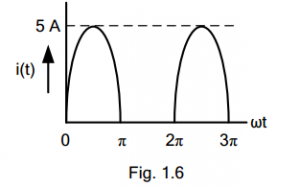A.  5√2

B.  2.5√2

C.  5/π

D.  0

Solution:

126.  A constant current of 2.8 exists in a resistor. The rms value of current is

A.  2.8 A

C.  1.4 A

D.  undefined

Solution:

127.  The rms value of a half-wave rectified symmetrical square wave  current of 2 A is

A.  √2  A

B.  1 A

C.   1/√ 2 A

D.  √3  A

Solution:

128.  The rms value of the voltage v(t) = 3 + 4 cos (3t) is

A.  √17 V

B.  5 V

C.  7 V

D.  (3 + 2√2 ) V

Solution:

129.  The rms value of the resultant current in a wire which carries a dc current of 10 A and a sinusoidal alternating current of peak value 20 A is

A.  14.1 A

B.  17.3 A

C.  22.4 A

D.  30.0 A

Solution:

130.  For the triangular waveform in the figure, the rms value of voltage is equal to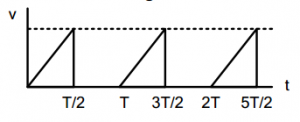A.  √(1/6) V

B.  √(1/3) V

C.  1/3 V

D.  √(2/3) V

Solution:

131.  The rms value of the periodic waveform given in the figure is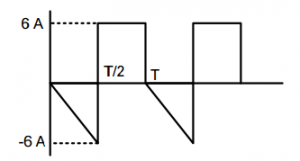A.   2√6  A

B.   6√2  A

C.  √(4/3)  A

D.  1.5 A

Solution:

132.  If i1 = 120 cos (100πt + 30°) and i2 = -0.1 cos (100πt + 100°) then i2 leads i1 by ____.

A.  -110 degrees

B.  60 degrees

C.  -60 degrees

D.  110 degrees

Solution:

133.  If v1 = sin (ωt + 30°) and v2 = -5 sin (ωt – 15°) then v1 leads v2 by ____.

A.  225 degrees

B.  30 degrees

C.  45 degrees

D.  none of these

Solution:

134.  The rms  value of a rectangular wave of period T, having a value of +V for a duration, T1 (<T) and –V for the duration T – T1 = T2 equals ____.

A.  V

B.  (T1 – T2)/T*V

C.  V/√2

D.  (T1/T2)* V

Solution:

135.  The rms value of the voltage waveform v(t) = sin 10t + sin 20t is ____.

A.  1

B.  1/2

C.  1/√2

D.  √2

Solution:

136.  For  the  voltage  waveform  v(t)  =  2  +  cos  (ωt  +  180°)  find  the  ratio  of Vrms/Vave.

A. 3/2√2

B.  √3/2

C.  π/2

D.  π

Solution:

137.  The rms value of the periodic wave form e(t) shown in the figure is _____.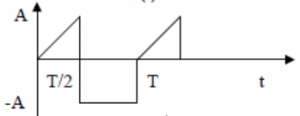A.   A√(3/2)     C.  A√(1/3)

B.   A√(2/3)     D.  A√2

Solution:

138.  Which of the waveforms are having unity peak factor?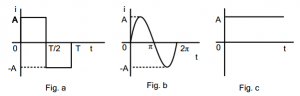A.  figure a and b

B.  figure b and c

C.  figure a and c

D.  none of these

Solution:

139.  The length of time between a point in one cycle to the same point of the next cycle of an AC wave is the ______.

A.  frequency

B.  period

C.  magnitude

D.  polarity

Solution:

140.  In an experiment, a sinusoidal wave form is observed to complete 8 cycles in 25 msec. Determine the frequency of the wave form.

A.  320 Hz

B.  40 Hz

C.  200 Hz

D.  64 Hz

Solution:

141.  If emf in a circuit is given by e = 100 sin 628t, the maximum value of voltage and frequency is ______.

A.  100 V, 50 Hz

B.  100 V, 100 Hz

C.   50√2  V, 50 Hz

D.   50√2  V, 100 Hz

Solution:

142.  A sinusoidal voltage wave has an RMS value of 70.71 V and a frequency of 60  Hz.  Determine  the  value  of  the  voltage  0.0014  second  after  the  wave crosses the ωt axis.

A.  70.71 V

B.  100 V

C.  50 V

D.  141.42 V

Solution:

143.  An  alternating  current  varying  sinusoidally  with  frequency  of  50  Hz  has  an RMS value of 20 A. At what time measured from the positive maximum value will the instantaneous current be 14.14 A?

A.  1/600 sec

B.  1/200 sec

C.  1/300 sec

D.  1/400 sec

Solution:

144.  The average value of the function i = 50 sin ωt + 30 sin 3ωt is equal to ______.

A.  31.8 A

B.  25 A

C.  38.2 A

D.  51.43 A

Solution:

145.  For 200 Vrms value triangular wave, the peak value is equal to ______.

A.  200 V

B.  222 V

C.  282 V

D.  346 V

Solution:

146.  Determine  the  rms  value  of  a  semi-circular  current  wave  which  has  a maximum value of A.

A.  0.816 A

B.  0.23 A

C.  0.866 A

D.  0.707 A

Solution:

147.  The rms value of a half-wave rectified current is 100 A. Its value for full-wave rectification would be ______ amperes.

A.  141.4 A

B.  200 A

C.  200/π A

D.  400/π A

Solution:

148.  A half-wave rectified sine wave has an average value of 100 amp. What is the effective value?

A.  157 A

B.  444 A

C.  70.71 A

D.  100

Solution:

149.  The form factor of a half-wave rectified alternating current is ______.

A.  1.11

B.  1.57

C.  1.73

D.  1.0

Solution:

150.  Three alternating currents are given by i1  = 141 sin (ωt + 45°) A; i2  = 30 sin (ωt  +  90°)  A;  i3  =  20  cos  (ωt  –  120°)  A.  Find  the  equation  of  the  resultant current.

A.  167.4 sin (ωt + 45.66°)

B.  74.6 sin ωt

C.  143.8 sin (ωt + 51.4°)

D.  64.7 sin (ωt – 30°)

Solution:

#### Questions and Answers in AC Circuits

Following is the list of practice exam test questions in this brand new series:

MCQ in AC Circuits
PART 1: MCQ from Number 1 – 50                  Answer key: included
PART 2: MCQ from Number 51 – 100              Answer key: included
PART 3: MCQ from Number 101 – 150            Answer key: included
PART 4: MCQ from Number 151 – 200            Answer key: included
PART 5: MCQ from Number 201 – 250             Answer key: included
PART 6: MCQ from Number 251 – 300             Answer key: included
PART 7: MCQ from Number 301 – 350             Answer key: included
PART 8: MCQ from Number 351 – 400             Answer key: included
PART 9: MCQ from Number 401 – 450             Answer key: included
PART 10: MCQ from Number 451 – 500           Answer key: included

PinoyBIX Engineering. © 2014-2020 All Rights Reserved | How to Donate? |